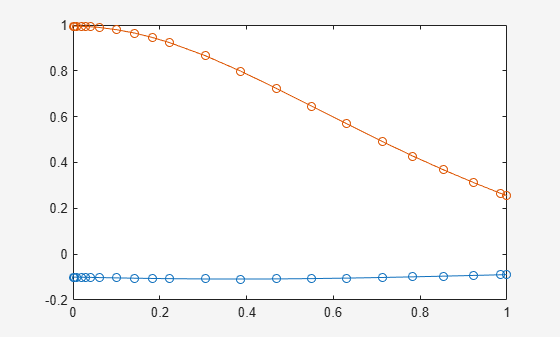# daeFunction

Convert system of differential algebraic equations to MATLAB function handle suitable for `ode15i`

## Syntax

``f = daeFunction(eqs,vars)``
``f = daeFunction(eqs,vars,p1,...,pN)``
``f = daeFunction(___,Name,Value)``

## Description

example

````f = daeFunction(eqs,vars)` converts a system of symbolic first-order differential algebraic equations (DAEs) to a MATLAB® function handle acceptable as an input argument to the numerical MATLAB DAE solver `ode15i`.```

example

````f = daeFunction(eqs,vars,p1,...,pN)` lets you specify the symbolic parameters of the system as `p1,...,pN`.```

example

````f = daeFunction(___,Name,Value)` uses additional options specified by one or more `Name,Value` pair arguments.```

## Examples

collapse all

Create the system of differential algebraic equations. Here, the symbolic functions `x1(t)` and `x2(t)` represent the state variables of the system. The system also contains constant symbolic parameters `a`, `b`, and the parameter function `r(t)`. These parameters do not represent state variables. Specify the equations and state variables as two symbolic vectors: equations as a vector of symbolic equations, and variables as a vector of symbolic function calls.

```syms x1(t) x2(t) a b r(t) eqs = [diff(x1(t),t) == a*x1(t) + b*x2(t)^2,... x1(t)^2 + x2(t)^2 == r(t)^2]; vars = [x1(t),x2(t)];```

Use `daeFunction` to generate a MATLAB® function handle `f` depending on the variables `x1(t)`, `x2(t)` and on the parameters `a`, `b`, `r(t)`.

`f = daeFunction(eqs,vars,a,b,r(t))`
```f = function_handle with value: @(t,in2,in3,param1,param2,param3)[in3(1,:)-param1.*in2(1,:)-param2.*in2(2,:).^2;-param3.^2+in2(1,:).^2+in2(2,:).^2] ```

Specify the parameter values, and create the reduced function handle `F` as follows.

```a = -0.6; b = -0.1; r = @(t) cos(t)/(1 + t^2); F = @(t,Y,YP) f(t,Y,YP,a,b,r(t));```

Specify consistent initial conditions for the DAE system.

```t0 = 0; y0 = [-r(t0)*sin(0.1); r(t0)*cos(0.1)]; yp0= [a*y0(1) + b*y0(2)^2; 1.234];```

Now, use `ode15i` to solve the system of equations.

`ode15i(F,[t0,1],y0,yp0)`Write the generated function handle to a file by specifying the `File` option. When writing to a file, `daeFunction` optimizes the code using intermediate variables named `t0`, `t1`, .… Include comments in the file using the `Comments` option.

Write the generated function handle to the file `myfile`.

```syms x1(t) x2(t) a b r(t) eqs = [diff(x1(t),t) == a*x1(t) + b*x2(t)^2,... x1(t)^2 + x2(t)^2 == r(t)^2]; vars = [x1(t), x2(t)]; daeFunction(eqs, vars, a, b, r(t), 'File', 'myfile')```
```function eqs = myfile(t,in2,in3,param1,param2,param3) %MYFILE % EQS = MYFILE(T,IN2,IN3,PARAM1,PARAM2,PARAM3) % This function was generated by the Symbolic Math Toolbox version 7.3. % 01-Jan-2017 00:00:00 YP1 = in3(1,:); x1 = in2(1,:); x2 = in2(2,:); t2 = x2.^2; eqs = [YP1-param2.*t2-param1.*x1;t2-param3.^2+x1.^2]; ```

Include the comment `Version: 1.1`.

```daeFunction(eqs, vars, a, b, r(t), 'File', 'myfile',... 'Comments','Version: 1.1');```
```function eqs = myfile(t,in2,in3,param4,param5,param6) ... %Version: 1.1 YP3 = in3(1,:); ...```

## Input Arguments

collapse all

System of first-order DAEs, specified as a vector of symbolic equations or expressions. Here, expressions represent equations with zero right side.

State variables, specified as a vector of symbolic functions or function calls, such as `x(t)`.

Example: `[x(t),y(t)]` or `[x(t);y(t)]`

Parameters of the system, specified as symbolic variables, functions, or function calls, such as `f(t)`. You can also specify parameters of the system as a vector or matrix of symbolic variables, functions, or function calls. If `eqs` contains symbolic parameters other than the variables specified in `vars`, you must specify these additional parameters as `p1,...,pN`.

### Name-Value Arguments

Specify optional pairs of arguments as `Name1=Value1,...,NameN=ValueN`, where `Name` is the argument name and `Value` is the corresponding value. Name-value arguments must appear after other arguments, but the order of the pairs does not matter.

Before R2021a, use commas to separate each name and value, and enclose `Name` in quotes.

Example: `daeFunction(eqns,vars,'File','myfile')`

Comments to include in the file header, specified as a character vector, cell array of character vectors, or string vector.

Path to the file containing generated code, specified as a character vector. The generated file accepts arguments of type `double`, and can be used without Symbolic Math Toolbox™. If the value is an empty character vector, `odeFunction` generates an anonymous function. If the character vector does not end in `.m`, the function appends `.m`.

By default, `daeFunction` with the `File` argument generates a file containing optimized code. Optimized means intermediate variables are automatically generated to simplify or speed up the code. MATLAB generates intermediate variables as a lowercase letter `t` followed by an automatically generated number, for example `t32`. To disable code optimization, use the `Optimize` argument.

Flag preventing optimization of code written to a function file, specified as `false` or `true`.

By default, `daeFunction` with the `File` argument generates a file containing optimized code. Optimized means intermediate variables are automatically generated to simplify or speed up the code. MATLAB generates intermediate variables as a lowercase letter `t` followed by an automatically generated number, for example `t32`.

`daeFunction` without the `File` argument (or with a file path specified by an empty character vector) creates a function handle. In this case, the code is not optimized. If you try to enforce code optimization by setting `Optimize` to `true`, then `daeFunction` throws an error.

Flag that switches between sparse and dense matrix generation, specified as `true` or `false`. When you specify `'Sparse',true`, the generated function represents symbolic matrices by sparse numeric matrices. Use `'Sparse',true` when you convert symbolic matrices containing many zero elements. Often, operations on sparse matrices are more efficient than the same operations on dense matrices.

## Output Arguments

collapse all

Function handle that can serve as input argument to `ode15i`, returned as a MATLAB function handle.

## Version History

Introduced in R2014b